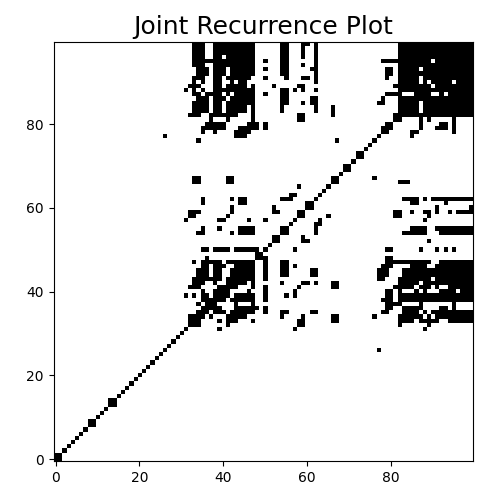# Joint Recurrence Plot¶

A joint recurrence plot is an extension of recurrence plots ( implemented as `pyts.image.RecurrencePlot`) for multivariate time series. A recurrence plot is built for each feature of the multivariate time series, then the set of recurrence plots is reduced to one single recurrence plot using the Hadamard product. This example illustrates this transformation. It is implemented as `pyts.multivariate.image.JointRecurrencePlot`.```# Author: Johann Faouzi <johann.faouzi@gmail.com>

import matplotlib.pyplot as plt
from pyts.multivariate.image import JointRecurrencePlot

X, _, _, _ = load_basic_motions(return_X_y=True)

# Recurrence plot transformation
jrp = JointRecurrencePlot(threshold='point', percentage=50)
X_jrp = jrp.fit_transform(X)

# Show the results for the first time series
plt.figure(figsize=(5, 5))
plt.imshow(X_jrp, cmap='binary', origin='lower')
plt.title('Joint Recurrence Plot', fontsize=18)
plt.tight_layout()
plt.show()
```

Total running time of the script: ( 0 minutes 0.327 seconds)

Gallery generated by Sphinx-Gallery